﻿ 当我有了超能力？！ | 蝴蝶停在猫耳上 | 橙光作品

——你这一声师父，难道是白叫的？

——我会在你身边。别怕。

——希望有一天，能带你去天柱峰顶看一看。

=·=·=·=·=·=·=·=·=·=·=·=·=·=·=·=·=·=·=·=·=·=

【更新信息】

【问题反馈方式】
①评论区@作者
②作者QQ：704946153
③作品交流群：206467309

=·=·=·=·=·=·=·=·=·=·=·=·=·=·=·=·=·=·=·=·=·=

【作品活动公告】

=·=·=·=·=·=·=·=·=·=·=·=·=·=·=·=·=·=·=·=·=·=

【特别注明】

=·=·=·=·=·=·=·=·=·=·=·=·=·=·=·=·=·=·=·=·=·=

【版权信息】

（所有主角、重要配角、UI等皆由画师和画师助手完成）
CV：Fú萝北（饰演肖不凡）

（如有遗漏请联系作者补充）
作品人气 15297
鲜花 5726
字数 197286

收藏 1714
发布时间 2018-09-27
最后更新 2019-10-07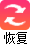• 作品角色
• 配音演员
• 完成度
• 试听•1
啵儿一个
•10
浪漫告白
•99
爱的尬舞
•520
橙光别墅

00 00 00 后可表白
•100
精美糖果
•200
香甜蛋糕
•600
诱人香槟
•1200
烛光晚餐

作品推荐领取成功！快去个人中心佩戴，ta可以帮你喜欢的作品吸引更多玩家！
作品简介 更新日志 投票
在一场车祸中离奇生还的沈浅熙，被告知有超能力的潜质。迷迷糊糊报考进入超研学院，等待她的却是父母拒绝支付学费的窘境。
看似平凡，却有着惊人身世的同窗；披着的仙风道骨的外表，实则资深游戏宅的道长；冷若冰霜却意外体贴入微的对手。当这些人与沈浅熙的人生互相交错，当权术与阴谋逐渐逼近，死亡的阴影笼罩在所有学员头上，沈浅熙能否在这名唤“学园”的地狱中逃出生天。
踏向通往深渊的路途。
脚下是死亡的余烬。
权力、欲望、野心、争斗……
将会是谁，在迷雾之中握住你的手？

李青玄：这位道友，吃冰淇淋吗？
肖不凡：还真是个傻的。
星洛：换人不必。别添乱。

肖不凡：
——你这一声师父，难道是白叫的？

星洛：
——我会在你身边。别怕。

李青玄：
——希望有一天，能带你去天柱峰顶看一看。

=·=·=·=·=·=·=·=·=·=·=·=·=·=·=·=·=·=·=·=·=·=

【更新信息】
一周更新字数5000字（只多不少）
预计完结字数20万字（只多不少）
预计2020年2月完结
稳定更新，断更全花返还！！（3朵花=1彩虹币）

【问题反馈方式】
①评论区@作者
②作者QQ：704946153
③作品交流群：206467309
作品交流群：206467309 加群注明橙光昵称，欢迎进群讨论剧情^^！

=·=·=·=·=·=·=·=·=·=·=·=·=·=·=·=·=·=·=·=·=·=

【作品活动公告】
第三波活动
福利图活动：表白人气第一的角色，画师会以此角色画一张图以示感谢（按月结算，奖励图在群里发），快为你喜欢的角色打call吧♪(^∇^*)

长评活动：每月选5个精品长评送8彩虹币，其余长评送3W积分

关于打卡活动的所有问题请在群里艾特作者或者QQ私戳作者^^
可选择微信返现和彩虹币，完成活动后带截图私戳群主哦
作品交流群：206467309 加群注明橙光昵称，欢迎进群^^！

=·=·=·=·=·=·=·=·=·=·=·=·=·=·=·=·=·=·=·=·=·=

【特别注明】
该作品由『Galaxy工作室』齐心协力完成！
工作室成员：
江月；白衣；蝴蝶停在猫耳上；姜暖苏；十分；猴砸；Fú萝北；浮殊；秀花；沫子；老符离

=·=·=·=·=·=·=·=·=·=·=·=·=·=·=·=·=·=·=·=·=·=

【版权信息】
统筹/策划：江月
剧本：姜暖苏；白衣；江月；十分
制作：蝴蝶停在猫耳上
画师：江月
画师助手：猴砸
（所有主角、重要配角、UI等皆由画师和画师助手完成）
CV：Fú萝北（饰演肖不凡）
浮殊（饰演水涼）
老符离
宣传：秀花
策划：江月
魔法转场：橙光正版素材库入驻素材（作者：莳陌）
紫金粒子转场：橙光正版素材库入驻素材（作者：柔树）
闪金光效转场：橙光正版素材库入驻素材（作者：天天天天黑）
其余素材均来自橙光正版素材库
（如有遗漏请联系作者补充）

还没创建过更新日志

如果您是作者，登录后可以在此创建更新日志。作品更新动态可以写在这里啦~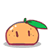正在加载~

### 作者菌哟，赶快到【作品投票设置】区为此作品设置投票吧！全部评论(0) 精品评论(0)
发表评论 可以用 @ 召唤你的小伙伴哦~ 个字

举报评论

请选择举报原因

×

提交

取消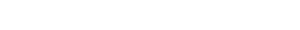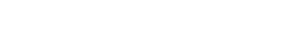您还未进行实名认证。
请完成实名认证后再发布评论。

将该精评分类至

鲜花榜 谁来看过
总榜 周榜

本作品由 蝴蝶停在猫耳上 制作，尚未经过审核，请勿传播。

作品不能赠送鲜花，若作品内存在敏感内容，请及时举报。

已帮您收藏该作品，可通过“我的收藏”查看该作品。
确定
/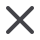### 最近7日榜 最近7日榜统计鲜花应援和投票应援 总排行榜 总榜统计鲜花应援和投票应援 作品总排行榜 统计角色卡在作品下的星运值这里空空如也，快来抢先当第1位